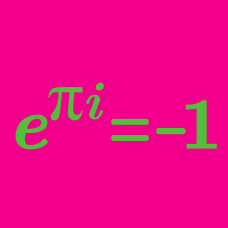Algebra

# De Moivre's Theorem: Level 3 Challenges

$\large { z }^{ 3 }=1$

What is the set of all $z$ that satisfy the equation above?

Note: $\omega = \frac{ -1 + \sqrt 3 i}{2}$ where $i =\sqrt{-1}$.

Find the value of $(2-\omega)(2-\omega^2)(2-\omega^{10})(2-\omega^{11}).$

Details and Assumptions:

$\omega$ is a non-real cube root of unity.

The five roots of the equation $z^{5}=4-4i$ each take the form

$\Large \sqrt{2} e ^ { \frac{ k \pi i } { 20} },$

where $k$ is a positive integer less than 40.

Find the sum of all values of $k$.

$\large x + \frac 1 x = \sqrt 3\ , \ \ \ \ \ \ \ x^{200} + \frac {1}{x^{200}} = \ ?$

If the $6$ solutions of $x^{6}=-64$ are written in the form $a+ib$, where $a$ and $b$ are real, then what is the product of those solutions with $a>0$?

×